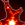# Reaction-diffusion systems and Turing patterns¶

In this tutorial, we will look at a simulation of a reaction-diffusion system described by a discretized partial differential equation used as a simple model animal coat pattern formation. The use of reaction–diffusion systems to explain pattern formation in biology may be traced back to Alan Turing's seminal 1952 paper (The Chemical Basis of Morphogenesis).

We will use HoloViews to investigate how the simulated reaction-diffusion system evolves over time for one initial state and then again over varying values of one of the simulation parameters. In the process, it will become clear how easy it is to leverage HoloViews to explore the behaviour of existing analysis or simulation code.

The simulation code for this tutorial has been adapted directly from the freely available recipe in the IPython Interactive Computing and Visualization Cookbook by Cyrille Rossant:As in the original tutorial, we first need to define a discretization of the Laplacian operator:

In :
import numpy as np

def laplacian(Z, dx):
"""
Function to computes the discrete Laplace operator of
a 2D variable on the grid (using a five-point stencil
finite difference method.)
"""
Ztop = Z[0:-2,1:-1]
Zleft = Z[1:-1,0:-2]
Zbottom = Z[2:,1:-1]
Zright = Z[1:-1,2:]
Zcenter = Z[1:-1,1:-1]
return (Ztop + Zleft + Zbottom + Zright - 4 * Zcenter) / dx**2


Now we can refactor the code from the original tutorial into a function that simulates the reaction-diffusion process. Note that this version uses a coarser time-step and returns copies of the reaction state over time as a list of (time, np.array) tuples:

In :
def reaction_diffusion(a=2.8e-4, b=5e-3, tau=0.1, k=-0.005, samples=10):
"""
We simulate the PDE with the finite difference method.

The samples value is the number of equally spaced samples
to collect over the total simulation time T.
"""
size = 100         # size of the 2D grid
dx = 2./size       # space step
T = 10.0           # total time
dt = 4.5 * dx**2    # simulation time step
n = int(T/dt)

result = []
U = np.random.rand(size, size)
V = np.random.rand(size, size)

sample_times = [int(el) for el in np.linspace(0, n, samples)]

for i in range(n):
# We compute the Laplacian of u and v.
deltaU = laplacian(U, dx=dx)
deltaV = laplacian(V, dx=dx)
# We take the values of u and v inside the grid.
Uc = U[1:-1,1:-1]
Vc = V[1:-1,1:-1]
# We update the variables.
U[1:-1,1:-1], V[1:-1,1:-1] = \
Uc + dt * (a * deltaU + Uc - Uc**3 - Vc + k), \
Vc + dt * (b * deltaV + Uc - Vc) / tau
# Neumann conditions: derivatives at the edges
# are null.
for Z in (U, V):
Z[0,:] = Z[1,:]
Z[-1,:] = Z[-2,:]
Z[:,0] = Z[:,1]
Z[:,-1] = Z[:,-2]

if i in sample_times:
result.append((i * dt,U.copy()))
return result


The array U represents the concentration of some compound involved in pigmentation, whereas the V array represents some other substance that reacts with the first compound to impede pigmentation. The partial differential equation for the evolution of these two compounds over time may be described by these two coupled equations:

$$\frac{\partial u}{\partial t} = a \Delta u + u - u^3 - v + k$$$$\tau \frac{\partial v}{\partial t} = b \Delta v + u - v$$

## Visualizing the simulation with HoloViews¶

The code above is a fairly direct translation of the original recipe except we now collect the simulation results over time as numpy arrays instead of simply mutating the U array until the final state is reached.

To visualize the results of the simulation using the default parameter, we first load the ipython extension and import the HoloMap and Image classes:

In :
import holoviews as hv
hv.notebook_extension()HoloViewsJS successfully loaded in this cell.

Lets run the first simulation which should complete after a few seconds:

In :
sim1 = reaction_diffusion()


Using a HoloMap of Image elements, we can view the evolution of our reaction-diffusion process over time (using the 'copper' color map). All we need to do is built a dictionary of Image elements indexed over time and pass it to the HoloMap where we declare the key dimension as 'Time':

In :
%%opts Image (cmap='copper')
hv.HoloMap({time: hv.Image(array) for (time, array) in sim1}, kdims=['Time'])

Out:

This shows how the reaction-diffusion pattern self-organizes from a random initial state when the default parameter values are used. In the original recipe, only a single frame displaying the final state was shown but using HoloViews we can easily view a 3-dimensional space (two spatial dimensions and time).

## Visualizing more dimensions¶

We can now go further and visualize the system in higher-dimensional spaces if we wish. Here we will examine a 4-dimensional space by exploring small variations in the 'a' parameter in addition to the 3-dimensions we explored above:

In :
a_values = np.linspace(2.8e-4, 6e-4, 3)


Again we collect the results across our three simulations into a list, keeping track of the associated time and 'a' parameter with each numpy array:

In :
sim2 = [((time, a_value), array) for a_value in a_values
for (time, array)  in reaction_diffusion(a=a_value)]


Now we build our second example of a HoloMap use a dictionary of Image elements. This time the keys are 2-tuple containing the corresponding values of the 'Time' and 'a' dimensions. These two dimensions are declared to the HoloMap as key_dimensions:

In :
%%opts Image (cmap='copper')
hv.HoloMap({key: hv.Image(array) for (key,array) in sim2}, kdims=['Time', 'A'])

Out: